# Community

Blog Events Webinars Tutorials Forum
×
Community Blog Ideas for Using Recursive Autoencoders for Intelligent Layout Generation

# Ideas for Using Recursive Autoencoders for Intelligent Layout Generation

This article uses the main ideas of the "READ: Recursive Autoencoders for Document Layout Generation" paper and discusses its implementation in frontend scenarios.

By Queyue## Overview

The layout is important in some design and terminal display scenarios. In a design layout, we first classify basic elements into certain categories based on the scenario. For example, we can classify elements as images and text, and then classify text elements as titles and paragraphs. Each element will have a corresponding position in this design document. Building on some recent research on sample generation and enhancement, the design of models to automatically generate layouts has become a popular area of research.

The READ: Recursive Autoencoders for Document Layout Generation paper combined a recursive neural network (RvNN) and an autoencoder to automatically generate different layouts from random Gaussian distributions. It also introduced a combined measurement method to measure the method quality. The following sections describe the methods used in this paper and some ideas for implementing these methods in frontend scenarios. If you are interested in this paper, click here to read it.

## RvNN and Autoencoder

First, I will give a simple introduction to the RvNN and variational autoencoder (VAE) technologies. For more information about these two technologies, search for the relevant materials on the Internet.

### RvNN

At first glance, you may confuse an RvNN with a recurrent neural network (RNN) because both of them can be called RNN and can process variable-length data. However, these networks are based on different ideas. An RvNN essentially solves whether the sample space contains a tree or graph structure.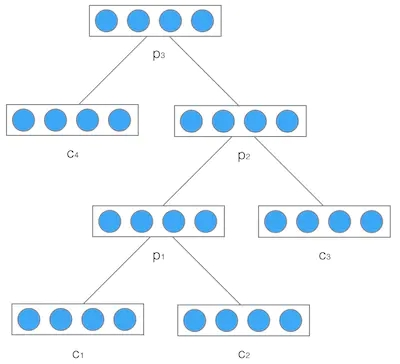As shown in the preceding figure, each input of a neural network contains two vectors, which we call child vectors. After the network performs a forward operation, the two child vectors form a parent vector. The parent vector then enters the network with another child node to generate a vector. This forms a recursive process. Finally, a root node or root vector is generated.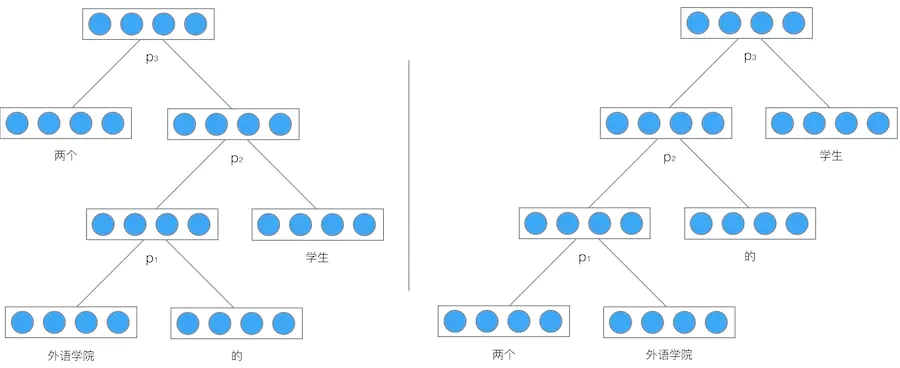If each leaf node in the preceding example is a word expression, the resulting trained network can merge these words into a vector in the semantic space. The vector can represent the corresponding sentence, and similar vectors can express the same semantics.

### VAE

A VAE is a type of autoencoder. Those who know about generative adversarial networks (GANs) will be familiar with VAEs. First, I will give a brief introduction to autoencoders.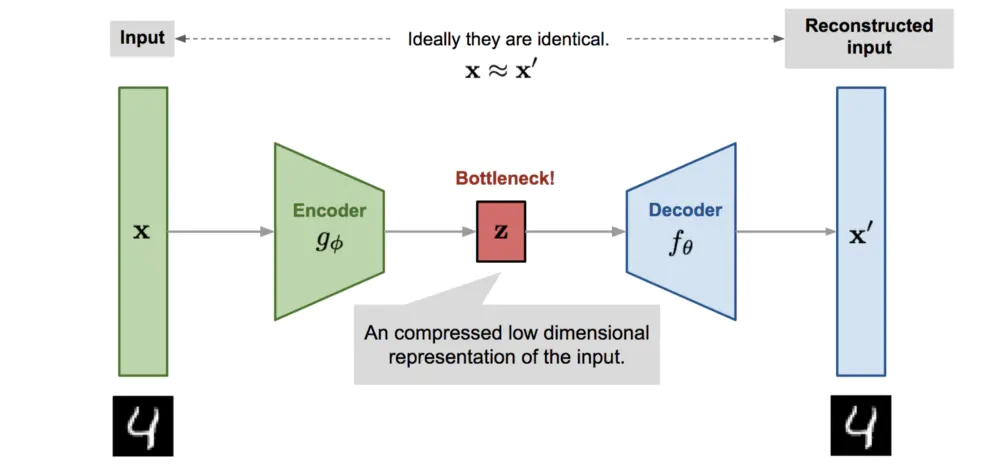Let°Øs say you have two networks. The first network maps a high-dimensional vector x to a low-dimensional vector z. In the preceding figure, we map an image to a one-dimensional vector. Then, the second network maps vector z to a high-dimensional vector x1. Our training target is to make x and x1 as close as possible. This way, we can regard vector z as a representation of x. The trained x1 is a generative network and can generate the image represented by z.

VAEs have made some improvements to normal autoencoders. They allow the encoder to generate an extra random vector, which we call the variance. The variance and z are combined as the input for the next step, ensuring a more stable model.

## Training Data Representation

To automatically generate layouts, we need a data representation method. In this search, our original data is design documents and the classification, position, and size of each basic element in these documents, which are given by annotations. To train the RvNN, we need to structurally disassemble these annotations and convert them into training data.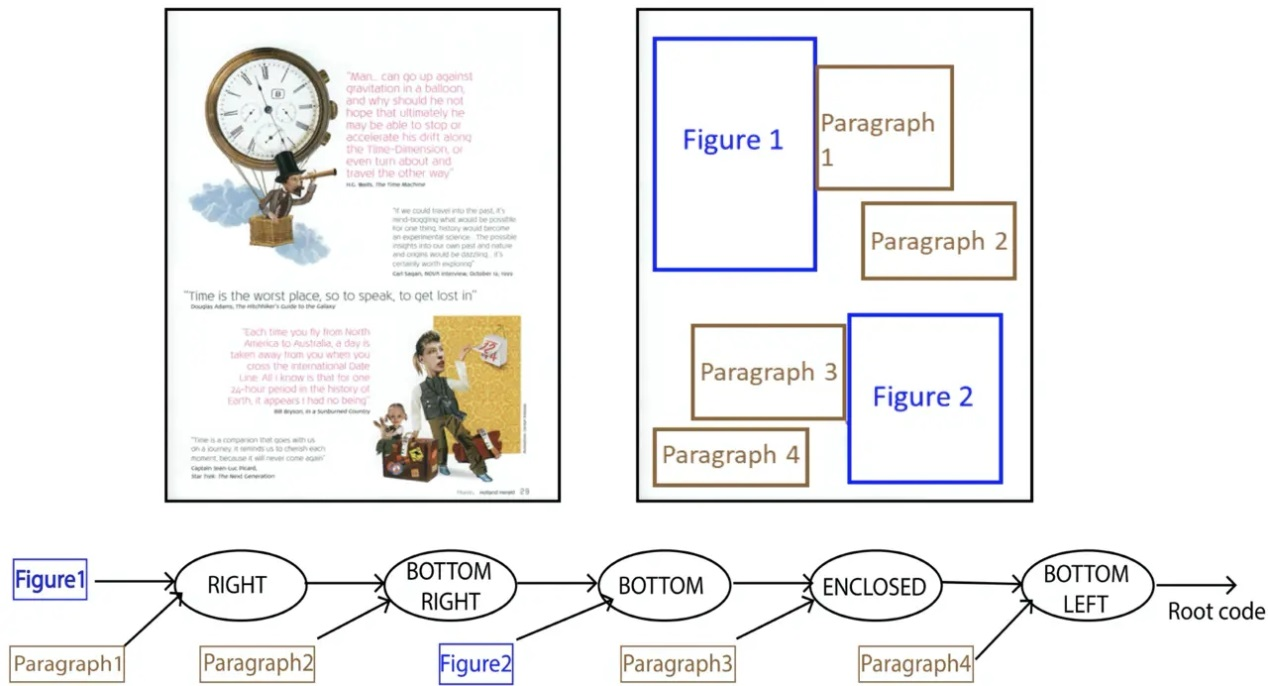As shown in the preceding figure, to better adapt the data to the RvNN, we convert it into a binary tree. Then, we scan the design document from left to right and from top to bottom. Each basic element is a leaf node. The nodes are merged from the bottom up based on the scanning sequence. We call merged nodes internal nodes, and these are ultimately merged into a root node. Each internal node has relative position information that is the relative position of the two merged elements. This information can be classified into right, bottom right, bottom, enclosed, and bottom left. The width and height of the bounding box for each leaf node are first normalized to [0, 1].

## Building a Recursive Model

For the training data, we generate a vector for each left node based on its width, height, and classification information. The width and height values are between [0, 1], and the classification is a one-hot vector. Then, we use a single-layer neural network to map the generated vector into a dense vector n-D. The empirical value of n introduced in the paper is 300. This way, the input is a 300-dimensional vector.

### SRE

The Spatial Relationship Encoder (SRE) in an RvNN is a multi-layer perceptron. In the paper, the perceptron only has one or two hidden layers. The input of this perceptron is two nodes, and the output is the merged node.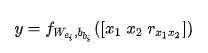As shown in the preceding formula, x1 and x2 are the n_D vectors of the two input nodes, and r represents the relative position of the two vectors, with the element on the left as the reference. f expresses the multi-layer perceptron of the current SRE. After continuous encoding in the RvNN, a vector that represents the root node is generated.

### SRD

The Spatial Relationship Decoder (SRD) performs the reverse process of the SRE. It disassembles the parent node into two nodes.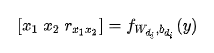During recursion, SREs and SRDs can use the same model structure. For example, we can classify SREs and SRDs into different types based on their relative position type and use the same network to train SREs and SRDs of the same type. In addition, we can train a neural network to determine whether a node is an internal node or a leaf node. If it is an internal node, we will continue to decode it. If it is a leaf node, we will map the node to the bounding box and classification of the element.

## Model Training

After a model is determined, we will define the loss function for model training. The following figure shows the expression: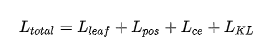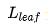indicates the reconstruction error of a leaf node, which is the difference between the initial and decoded vectors of the leaf node.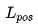indicates the reconstruction error of the relative position, which is the difference between the initial and decoded relative positions.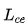indicates the classification loss of a relative position type, which is a standard cross-entropy loss function.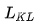indicates a Kullback-Leibler (KL) divergence between the space p(z) represented by the vector of the final root node and the standard Gaussian distribution q(z). Whenever possible, we want the vector of the root node to be a Gaussian distribution because we want the input of the SRD to be a random vector from the Gaussian distribution sample, so the model can automatically generate a layout.

I will not give a detailed description of the formulas for the preceding four loss functions here. Now, we can start to train the model based on the synthetic loss function.

## Model Measurement

The paper provides a measurement method called DocSim, which is used to measure the similarity between documents. This method is based on the BLEU method, which is a solution used to measure the translation system. DocSim compares two given documents: D and D'. For any pair of bounding boxes B and B' that are members of D and D', respectively, we assign a metric to measure the similarity between B and B' in terms of shape, position, and classification. The final measurement score of D and D' is the total weight of the metrics assigned for many sets of bounding boxes.

## Experimental Datasets

### ICDAR2015 Dataset

The ICDAR2015 dataset is a public dataset that contains 478 design documents. The design documents are mainly magazine articles. For these documents, we consider the title, paragraph, footer, page number, and graphic semantic categories.

### User-Solicited (US) Dataset

The US dataset contains 2036 design documents. These design documents mainly contain expression data submitted by users, including tax forms and bank applications. These files usually feature complex structures and a large number of atomic element types. These features present a challenge for generating models.

## Experimental Results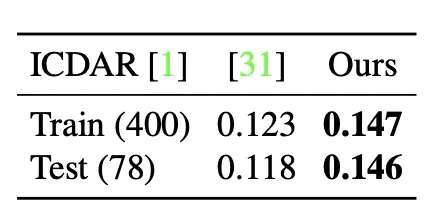The preceding figure shows the results of a comparison between the method proposed in the paper and the probabilistic approach in the ICDAR dataset. The value indicates the latent distribution similarity. According to the value, the method proposed in the paper is better.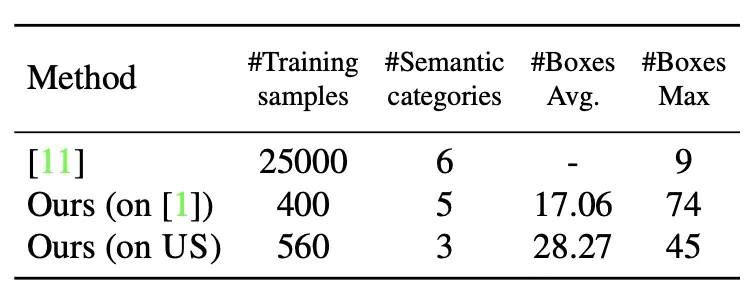The preceding figure shows the results of a comparison between the method proposed in the paper and LayoutGAN. The method proposed in the paper requires less training data samples and generates more elements.

## Some Ideas

The method provided in the paper is used to generate layouts. Frontend scenarios also involve conversion from an original design document to suitable layouts. For example, when we have an original image, we want to correctly extract basic elements in the image, determine the type of each element (image or text), and the element position.

Undoubtedly, research in this field requires a large amount of training data. If the solution proposed in the paper is applied to data in frontend scenarios, it could provide a sample generation and enhancement method, allowing us to obtain reasonable training data. Later, we will do additional research to verify the feasibility of the solution.

0 0 0
Share on

# Related Products

• ##Machine Learning Platform For AI

An end-to-end platform that provides various machine learning algorithms to meet your data mining and analysis requirements.

• ##Intelligence Brain Solution

ET Brain is Alibaba Cloud’s ultra-intelligent AI Platform for solving complex business and social problems

• ##Epidemic Prediction Solution

This technology can be used to predict the spread of COVID-19 and help decision makers evaluate the impact of various prevention and control measures on the development of the epidemic.

• ##mPaaS

Help enterprises build high-quality, stable mobile apps

More Posts by Alibaba F(x) Team